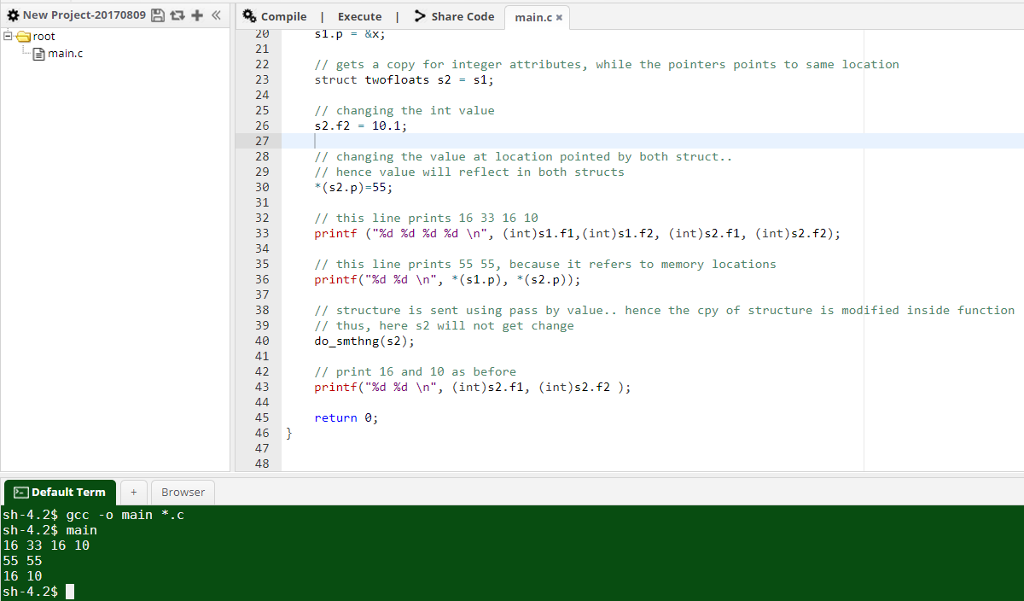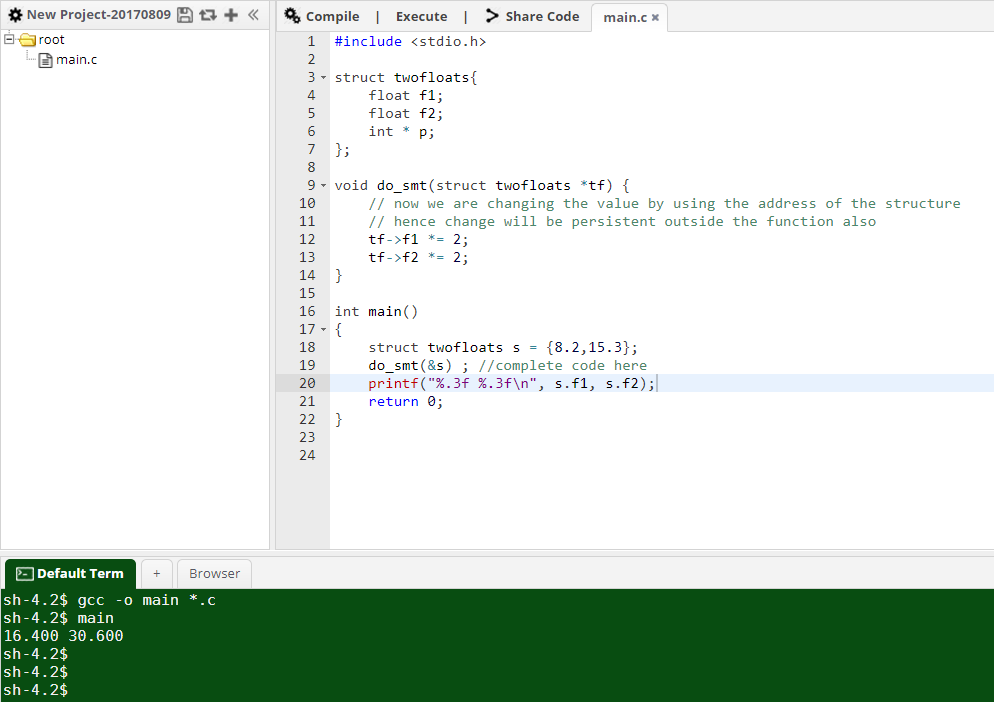# Question & Answer: C program, pointers…..

C program, pointers

1.
Struct twofloats{

Don't use plagiarized sources. Get Your Custom Essay on
Question & Answer: C program, pointers…..
GET AN ESSAY WRITTEN FOR YOU FROM AS LOW AS \$13/PAGE

Float f1;
Float f2:
Int * p;

}

Void do_smthng(struct twofloats tf) {

tf.f1 = 0.0;
tf.f2 = 1.0;
}

Main(){
struct twofloats s1 = {16.65,33.3};
Int x = 5;
s1.p = &x;
struct twofloats s2 = s1;
s2.f2 = 10.1;
*(s2.p)=55;
printf (“%d %d %d %d n”, (Int)s1.f1,(Int)s1.f2, (Int)s2.f1, (Int)s2.f2);

printf(“%d %d n”, *(s1.p), *(s2.p));

Do_smthng(s2);

Printf(“%d %d n”, (Int)s2.f1, (Int)s2.f2 l;

//what are the outputs of printf, do_smth?

2.
Complete program so output is 16.400, 30.600 double the field values

Struct twofloats{
Float f1;
Float f2;
}

// complete code below
Void do_smt(   ) {

}

main() {

struct twofloats s = {8.2,15.3};

do_smt(   ) ; //complete code here

Printf(“%.3f %.3fn”, s.f1, s.f2);

Question 1:

#include <stdio.h>

struct twofloats{
float f1;
float f2;
int * p;
};

void do_smthng(struct twofloats tf) {
// it is a pass by value struct
// hence the original structure will not get change when we change values here
tf.f1 = 0.0;
tf.f2 = 1.0;
}

int main()
{
struct twofloats s1 = {16.65,33.3};
int x = 5;
s1.p = &x;

// gets a copy for integer attributes, while the pointers points to same location
struct twofloats s2 = s1;

// changing the int value
s2.f2 = 10.1;

// changing the value at location pointed by both struct..
// hence value will reflect in both structs
*(s2.p)=55;

// this line prints 16 33 16 10
printf (“%d %d %d %d n”, (int)s1.f1,(int)s1.f2, (int)s2.f1, (int)s2.f2);

// this line prints 55 55, because it refers to memory locations
printf(“%d %d n”, *(s1.p), *(s2.p));

// structure is sent using pass by value.. hence the cpy of structure is modified inside function
// thus, here s2 will not get change
do_smthng(s2);

// print 16 and 10 as before
printf(“%d %d n”, (int)s2.f1, (int)s2.f2 );

return 0;
}Question 2′;

#include <stdio.h>

struct twofloats{
float f1;
float f2;
int * p;
};

void do_smt(struct twofloats *tf) {
// now we are changing the value by using the address of the structure
// hence change will be persistent outside the function also
tf->f1 *= 2;
tf->f2 *= 2;
}

int main()
{
struct twofloats s = {8.2,15.3};
do_smt(&s) ; //complete code here
printf(“%.3f %.3fn”, s.f1, s.f2);
return 0;
}